Courses

# Plain Carbon Steel, Iron Carbon Equilibrium Diagram & Cast Iron - MCQ Test 1

## 20 Questions MCQ Test RRB JE for Mechanical Engineering | Plain Carbon Steel, Iron Carbon Equilibrium Diagram & Cast Iron - MCQ Test 1

Description
This mock test of Plain Carbon Steel, Iron Carbon Equilibrium Diagram & Cast Iron - MCQ Test 1 for Mechanical Engineering helps you for every Mechanical Engineering entrance exam. This contains 20 Multiple Choice Questions for Mechanical Engineering Plain Carbon Steel, Iron Carbon Equilibrium Diagram & Cast Iron - MCQ Test 1 (mcq) to study with solutions a complete question bank. The solved questions answers in this Plain Carbon Steel, Iron Carbon Equilibrium Diagram & Cast Iron - MCQ Test 1 quiz give you a good mix of easy questions and tough questions. Mechanical Engineering students definitely take this Plain Carbon Steel, Iron Carbon Equilibrium Diagram & Cast Iron - MCQ Test 1 exercise for a better result in the exam. You can find other Plain Carbon Steel, Iron Carbon Equilibrium Diagram & Cast Iron - MCQ Test 1 extra questions, long questions & short questions for Mechanical Engineering on EduRev as well by searching above.
QUESTION: 1

Solution:

Ans. (c)

QUESTION: 2

Solution:

Ans. (d)

QUESTION: 3

### Match List I (Alloy) with List II (Application) and select the correct answer using the codes given below the Lists: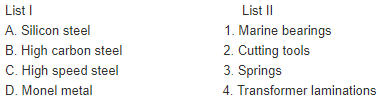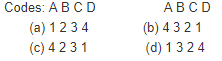Solution:

Ans. (b)

QUESTION: 4

For the pipe fitting like elbow, tee, union etc. which of the following is preferred?

Solution:

Ans. (b)

QUESTION: 5

Consider the following statements:
Strength of steel increases with carbon content.Young's Modulus of steel increases with carbon content.Young's Modulus of steel remains unchanged with variation of carbon content.Which of the statements given above is/are correct?

Solution:

Ans. (c)

QUESTION: 6

Presence of hydrogen in steel causes

Solution:

Ans. (c)

QUESTION: 7

Match List I (Percentage of carbon content in plain carbon steel) with List II (Application) and select the correct answer using the codes given below the lists: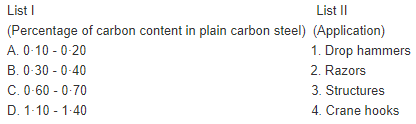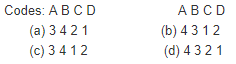Solution:

Ans. (a)

QUESTION: 8

Match List-I (Fe-Fe3C Phase Diagram Characteristic) with List-II (Phase) and select the correct answer using the code given below the Lists: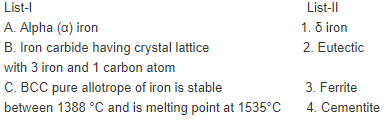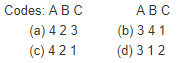Solution:

Ans. (b)

QUESTION: 9

Consider the following statements:
1. From design considerations, it is always advantageous to place cast iron ribs on thetension side rather than on the compression side.
2. Cast iron is an excellent choice for machine tool guides and frames.
3. Cast iron parts have low notch sensitivity.
Which of these statements are correct?

Solution:

Ans. (b)
Since cast iron is strong in compression and weak in tension. Therefore,
statement 1 is wrong.

QUESTION: 10

Which of the following display properties similar to that of steel
1. Black-heart cast iron
2. White-heart cast iron
3. Gray cast iron
4. Pig iron

Solution:

Ans. (a)

QUESTION: 11

Assertion (A): Fracture surface of grey cast iron is dark.
Reason (R): Failure takes place along the weak cementite plates.

Solution:

(a) Both A and R are true. Also R gives satisfactory explanation for A.

QUESTION: 12

Addition of magnesium to cast iron increases its

Solution:

Ans. (b)

QUESTION: 13

Eutectoid reaction occurs at

Solution:
QUESTION: 14

An iron-carbon binary alloy has 0.5% C by weight. What is this alloy called?

Solution:

Ans. (c)

QUESTION: 15

Match List I with List II and select the correct answer using the codes given below the lists: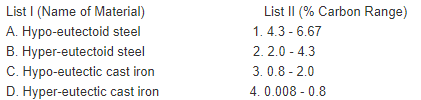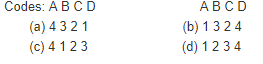Solution:

Ans. (a)

QUESTION: 16

Consider the following statements:
Addition of silicon to cast iron
1. Promotes graphite module formation.
2. Promotes graphite flake formation.
3. Increases the fluidity of the molten metal.
4. Improves the ductility of cast iron.
Select the correct answer using the codes given below:

Solution:

Ans. (b)

QUESTION: 17

Assertion (A): Cast iron is generally hard, brittle and wear resistant.
Reason (R): Cast iron contains more than 2% carbon and as such the percentagecementite in it is higher.

Solution:

Ans. (a)

QUESTION: 18

Nodular grey cast iron is obtained from the grey cast iron by adding a smallamount of

Solution:

Ans. (c)

QUESTION: 19

Machine tool manufacturers prefer grey cast-iron grade 40 for producing machinecolumns and tables because grey cast-iron is
1. Heavy
2. Easily castable
3. Easily weldable
4. Having good damping capacity
Select the correct answer using the codes given below:
Codes:

Solution:

Ans. (b)

QUESTION: 20

Consider the following statements:
1. Cast Iron has poor ability to damp vibrations.
2. Cast Iron has higher compressive strength compared to that of steel.
3. Cast Iron parts are suitable where permanent deformation is preferred over fracture.
Which of the statements given above is/are correct?

Solution:

Ans. (d)